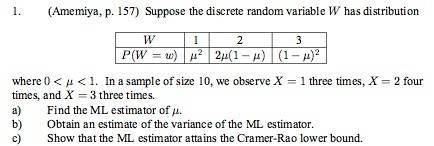# Maximum Likelihood Estimators

Scootertaj## Homework Equations

$$L(x,p) = \prod_{i=1}^npdf$$
$$l= \sum_{i=1}^nlog(pdf)$$
Then solve $$\frac{dl}{dp}=0$$ for p (parameter we are seeking to estimate)

## The Attempt at a Solution

I know how to do this when we are given a pdf, but I'm confused how to do this when we have a sample.

Homework Helper
Dearly Missed## Homework Equations

$$L(x,p) = \prod_{i=1}^npdf$$
$$l= \sum_{i=1}^nlog(pdf)$$
Then solve $$\frac{dl}{dp}=0$$ for p (parameter we are seeking to estimate)

## The Attempt at a Solution

I know how to do this when we are given a pdf, but I'm confused how to do this when we have a sample.

Are there no similar examples solved in your textbook or course notes? Could you find nothing at all on-line?

RGV

Scootertaj
Not so far, though I'll be talking to a graduate TA about it.